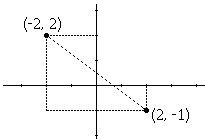# The Distance Formula

Very often, especially when measuring the distance in the plane, we use the formula for the Euclidean distance. According to the Euclidean distance formula, the distance between two points in the plane with coordinates (x, y) and (a, b) is given by

dist((x, y), (a, b)) = (x - a)² + (y - b)²

As an example, the (Euclidean) distance between points (2, -1) and (-2, 2) is found to be

 dist((2, -1), (-2, 2)) = √(2 - (-2))² + ((-1) - 2)² = √(2 + 2)² + (-1 - 2)² = √(4)² + (-3)² = √16 + 9 = √25 = 5.

The source of this formula is in the Pythagorean theorem. Look at the diagramThe horizontal distance between the points is 4 and the vertical distance is 3. Let's introduce one more point (-2, -1). With this small addition we get a right-angled triangle with legs 3 and 4. By the Pythagorean theorem, the square of the hypotenuse is (hypotenuse)² = 3² + 4². Which gives the length of the hypotenuse as 5, same as the distance between the two points according to the distance formula. This is of course always the case: the straight line segment whose length is taken to be the distance between its endpoints always serves as a hypotenuse of a right triangle (in fact, of infinitely many of them. We just chose the most convenient one.)

How good is the (Euclidean) distance formula for measuring real distances? This depends on the circumstances. In the plane - since the Earth is round, this means within relatively small areas of Earth's surface - it is pretty good, provided the distance is exactly what you want to estimate. If the question is, How fast you can get from one point to another while moving at a given speed, the Euclidean formula may not be very useful providing the answer. Indeed, in a city - just to take one example - it is often impossible to move from one point straight to another. There are buildings, streets busy with traffic, fences and what not, to be accounted for. In a city, one often finds that the taxicab distance formula

dist((x, y), (a, b)) = |x - a| + |y - b|

is more useful. In mathematics, the Euclidean distance is most fundamental. As one of the mechanical proofs of the Pythagorean theorem shows, the same is also true in physics, although in either science it's not the only distance formula used.### Taxicab/City-block Distance.Copyright © 1996-2018 Alexander Bogomolny

 65278934